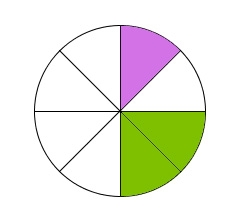# Unknown number

I think the number. I'll reduce it to its one-third. The result is then increased by one-third, and I get the number 12.

Correct result:

x =  35

#### Solution:

x/3+1/3= 12

x = 35

Our simple equation calculator calculates it.We would be pleased if you find an error in the word problem, spelling mistakes, or inaccuracies and send it to us. Thank you!Tips to related online calculators
Need help calculate sum, simplify or multiply fractions? Try our fraction calculator.
Do you have a linear equation or system of equations and looking for its solution? Or do you have quadratic equation?

## Next similar math problems:

• Forest nurseryIn the forest nursery after winter, they found that 1/10 stems died out of them. For them, they land 193 new spruces. How many spruces are in the forest nursery?
• Simple equationSolve the following simple equation: 2. (4x + 3) = 2-5. (1-x)
• Equation 29Solve next equation: 2 ( 2x + 3 ) = 8 ( 1 - x) -5 ( x -2 )
• Simple equation 1035= 7*3*x what is x?
• Mixed2improperWrite the mixed number as an improper fraction. 166 2/3
• One thirdIf 3/5 is 360, how much is 1/3?
• Find xSolve: if 2(x-1)=14, then x= (solve an equation with one unknown)
• Dropped sheetsThree consecutive sheets dropped from the book. The sum of the numbers on the pages of the dropped sheets is 273. What number has the last page of the dropped sheets?
• PearsThere were pears in the basket, I took two-fifths of them, and left six in the basket. How many pears did I take?
• Equation 15Solve equation with variables on both sides:
• EquationFind x: x + 1/2 = 1/3
• Unknown number 11That number increased by three equals three times itself?
• EqnSolve equation with fractions: 2x/3-50=40+x/4
• Grandmother and grandfatherGrandmother baked cakes. Grandfather ate half, then quarter of the rest ate Peter and Paul ate half of rest. For parents left 6 cakes. How many cakes maked the grandmother?
• TreesFrom the total number of trees in the orchard, there are two-fifths pearls and apples are three eighty. The rest of the trees are 9 ceremonial. How many trees are in the set?
• EquationSolve equation and check the result: 1.4x - 3/2 + x - 9,8 = x + 0,4/3 - 7 + 1,6/6
• NumberI think the number. If I add to its third seven I get same as when to its quarter add 8. Which is the number?